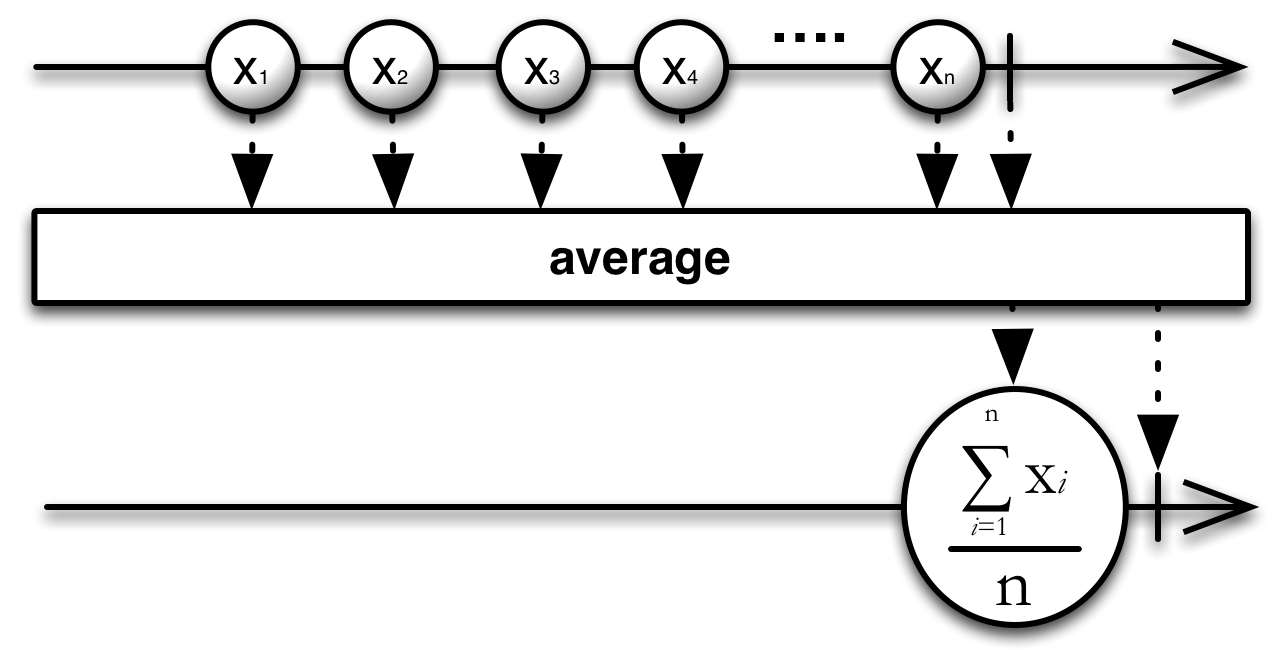/ReactiveX

# Average

calculates the average of numbers emitted by an Observable and emits this average

## Language-Specific Information

In RxGroovy, this operator is not in the ReactiveX core, but is part of the distinct `rxjava-math` module, where it is implemented with four type-specific operators: `averageDouble`, `averageFloat`, `averageInteger`, and `averageLong`. The following example shows how these operators work:

#### Sample Code

```def myObservable = Observable.create({ aSubscriber ->
if(false == aSubscriber.isUnsubscribed()) aSubscriber.onNext(4);
if(false == aSubscriber.isUnsubscribed()) aSubscriber.onNext(3);
if(false == aSubscriber.isUnsubscribed()) aSubscriber.onNext(2);
if(false == aSubscriber.isUnsubscribed()) aSubscriber.onNext(1);
if(false == aSubscriber.isUnsubscribed()) aSubscriber.onCompleted();
});

Observable.averageInteger(myObservable).subscribe(
{ println(it); },                  // onNext
{ println("Error encountered"); }, // onError
{ println("Sequence complete"); }  // onCompleted
);```
```2
Sequence complete```You can also average not the items themselves but the results of a function applied to each item, as in the illustration above, which emits the average number of sides on the figures emitted by the source Observable.

This operator will fail with an `IllegalArgumentException` if the source Observable does not emit any items.

This operator is not in the RxJava core, but is part of the distinct `rxjava-math` module, where it is implemented with four type-specific operators: `averageDouble`, `averageFloat`, `averageInteger`, and `averageLong`.You can also average not the items themselves but the results of a function applied to each item, as in the illustration above, which emits the average number of sides on the figures emitted by the source Observable.

This operator will fail with an `IllegalArgumentException` if the source Observable does not emit any items.RxJS implements this operator as average. The following code sample shows how to use it: Sample Code var source = Rx.Observable.range(0, 9).average(); var subscription = source.subscribe( function (x) { console.log('Next: ' + x); }, function (err) { console.log('Error: ' + err); }, function () { console.log('Completed'); }); Next: 4 CompletedYou can also average not the items themselves but the results of a function applied to each item, as in the illustration above, which emits the average number of sides on the figures emitted by the source Observable. Sample Code var arr = [ { value: 1 }, { value: 2 }, { value: 3 } ]; var source = Rx.Observable.fromArray(arr).average(function (x) { return x.value; }); var subscription = source.subscribe( function (x) { console.log('Next: ' + x); }, function (err) { console.log('Error: ' + err); }, function () { console.log('Completed'); }); Next: 2 Completed

`average` is found in the following distributions:

• `rx.all.js`
• `rx.all.compat.js`
• `rx.aggregates.js`

It requires one of the following:

• `rx.js`
• `rx.compat.js`
• `rx.lite.js`
• `rx.lite.compat.js`

© ReactiveX contributors
Licensed under the Apache License 2.0.
http://reactivex.io/documentation/operators/average.html